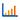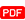# Analysis of Different Boundary Conditions on Homogeneous One-Dimensional Heat EquationTotal Views: 191 |Total Downloads: 215

## Authors

• Norazlina Subani Kolej GENIUS Insan, Universiti Sains Islam Malaysia, Bandar Baru Nilai, 71800, Nilai, Negeri Sembilan, Malaysia
• Muhammad Aniq Qayyum Mohamad Sukry Kolej GENIUS Insan, Universiti Sains Islam Malaysia, Bandar Baru Nilai, 71800, Nilai, Negeri Sembilan, Malaysia
• Muhammad Arif Hannan Kolej GENIUS Insan, Universiti Sains Islam Malaysia, Bandar Baru Nilai, 71800, Nilai, Negeri Sembilan, Malaysia
• Faizzuddin Jamaluddin Kolej GENIUS Insan, Universiti Sains Islam Malaysia, Bandar Baru Nilai, 71800, Nilai, Negeri Sembilan, Malaysia
• Ahmad Danial Hidayatullah Badrolhisam Kolej GENIUS Insan, Universiti Sains Islam Malaysia, Bandar Baru Nilai, 71800, Nilai, Negeri Sembilan, Malaysia

## Abstract

Partial differential equations involve results of unknown functions when there are multiple independent variables. There is a need for analytical solutions to ensure partial differential equations could be solved accurately. Thus, these partial differential equations could be solved using the right initial and boundaries conditions. In this light, boundary conditions depend on the general solution; the partial differential equations should present particular solutions when paired with varied boundary conditions. This study analysed the use of variable separation to provide an analytical solution of the homogeneous, one-dimensional heat equation. This study is applied to varied boundary conditions to examine the flow attributes of the heat equation. The solution is verified through different boundary conditions: Dirichlet, Neumann, and mixed-insulated boundary conditions. the initial value was kept constant despite the varied boundary conditions. There are two significant findings in this study. First, the temperature profile changes are influenced by the boundary conditions, and that the boundary conditions are dependent on the heat equation’s flow attributes.

## References

E. Agyeman and D. Folson, “Algorithm analysis of numerical solutions to the heat equation”, Int. J. of Comp. Appl., vol. 79 , pp. 975 – 8887, 2013.

A. A. Mamun, M. S. Ali and M. M. Miah, “A study on an analytical solution 1d heat equation of a parabolic partial differential equation and implement in computer programming”, Int. J. of Sci. and Eng. Res., vol. 9(9), pp . 2229 – 5518, 2018.

T. A. Biala and S. N. Jator, “A boundary value approach for solving three dimensional elliptic and hyperbolic partial differential equations”, SpringerPlus, vol. 4(588), 2015.

G. Papanikos and M. C. Gousidou-Koutita, “A computational study with finite element method and finite difference method for 2D elliptic partial differential equations”, Appl. Math., pp .1 – 21 , 2015.

N. Subani, F. Jamaluddin, M. A. H. Mohamed, and A. D. H. Badrolhisam, “Analytical solution of homogeneous one-dimensional heat equation with Neumann boundary conditions”, J. of Phys.: Conf. Ser., vol. 1551, pp. 1 – 13 , 2020.

S. Abarbanel, A. Ditkowski and B. Gustafsson, “On error bounds of finite di fference approximations to partial differential equations –Temporal behavior and rate of convergence”, J. of Sci. Comp., vol.15 , pp. 79-116, 2000.

M. A. Islam, S. M. K. Hossain and A. Rashid, “Numerical solution of one-dimensional heat equation by Crank Nicolson method”, Int. Conf. on Mechanical, Industrial and Energy Engineering, pp. 1 – 4, 2018.

B. Mebrate, “Numerical solution of a one dimensional heat equation with Dirichlet boundary conditions”, American. J. of Appl. Math., vol.3(6), pp . 305 – 311, 2015.

M. Roknujjaman and M. Asaduzzaman, “On the solution procedure of partial differential equation (PDE) with the methods of lines (MOL) using Crank-Nicholson method (CNM)”, American. J. of Appl. Math., vol. 6(1), pp . 1 – 7, 2018.

A. Tveito and R. Winther, Introduction to Partial Differential Equations: A Computational Approach, New York: Springer-Verlag, 1998.

J. Crank, The Mathematics of Diffusion 2nd Ed., London: Oxford University Press, Ely House, 1975.

S. Javed, Thermal Modelling and Evaluation of Borehole Heat Transfer, Sweeden: Chalmers University of Technology, 2012.

M. Sabaeian, H. Nadgaran and L. Mousave, “Analytical solution of the heat equation in a longitudinally pumped cubic solid-state laser”, Opt. Soc. of America, vol. 47(13), pp . 2317 – 2325, 2008.

A. Aidoo and M. Wilson, “A review of wavelets solution to stochastic heat equation with random inputs”, Appl. Math., vol. 6, pp. 2226 –2239, 2015.

J. As-Suyuti, Tafsir Jalalain versi Terjemahan Melayu oleh Bahrun Abu Bakar, Sinar Baru Algensindo, Bandung: Indonesia, 2003.

J. Al-Mahalli, Tafsir Jalalain versi Terjemahan Melayu oleh Bahrun Abu Bakar, Sinar Baru Algensindo, Bandung: Indonesia, 2003.

J. Al-Mahalli and J. As-Suyuti, Introduction to Tafsir Al-Jalalayn. Royal Aal al-Bayt Institute for Islamic Thought, Amman: Jordan, 2007.

G. Emenogu and N. Oko, “Numerical solution of parabolic initial-boundary value problem with Crank-Nicolson’s finite difference equations”, IOSR J. of Math., vol. 11(4), pp . 16 – 19 , 20

W. R. Gerald, Finite-Difference Approximations to the Heat Equation, Portland: Portland State University, 2011.

N. F. A. Jalil, Solving One Dimensional Heat Equation and Groundwater Flow Modeling Using Finite Difference Method, Malaysia: Universiti Teknologi Malaysia, 2011

S. R. Z. Zana, Numerical Solution of Diffusion Equation in One Dimension, North Cyprus: Eastern Mediterranean University, 2014.

V. Dabral, S. Kapoor and S. Dhawan, “Numerical simulation of one-dimensional heat equation: B-Spline finite element method”, Indian J. of Comp. Sci. and Eng., vol. 2(2), 2011.

C. Susan, L. Brenner and S. Ridgway, The Mathematical Theory of Finite Element Methods, 3rd Ed., Spain: Springer, 2008.

## Published

2021-03-30
CITATION
DOI: 10.33102/mjosht.v7i1.153
Published: 2021-03-30

## How to Cite

Subani, N. ., Mohamad Sukry, M. A. Q. ., Hannan, M. A. ., Jamaluddin, F. ., & Badrolhisam, A. D. H. . (2021). Analysis of Different Boundary Conditions on Homogeneous One-Dimensional Heat Equation. Malaysian Journal of Science Health & Technology, 7(1), 15–21. https://doi.org/10.33102/mjosht.v7i1.153

## Section

Integration of Science & Technology